# Prime ideal

## Definition

### Equivalent definitions in tabular format

No. Shorthand An ideal in a commutative unital ring is termed a prime ideal if ... An ideal$I$ in a commutative unital ring$R$ is termed a prime ideal if ...
1 product of two elements version It is a proper ideal and whenever the product of two elements in the ring lies inside that ideal, at least one of the elements must lie inside that ideal$I$ is proper in$R$ and whenever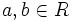$a, b \in R$ are (possibly equal, possibly distinct elements) such that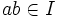$ab \in I$ then either$a \in I$ or$b \in I$ (note: it's also possible that both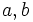$a,b$ are in$I$).
2 product of two ideals version It is a proper ideal and whenever the product of two ideals is contained in it, one of the ideals is contained in it.$I$ is proper in$R$ and whenever$J$ and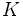$K$ are (possibly equal, possibly distinct) ideals such that$JK \subseteq I$, then either$J \subseteq I$ or$K \subseteq I$ (note: it's also possible that both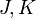$J,K$ are in$I$).
3 product of finitely many elements version It is a proper ideal and whenever the product of finitely many elements in the ring lies inside that ideal, at least one of the elements must lie inside that ideal$I$ is proper in$R$ and whenever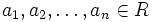$a_1,a_2,\ldots,a_n \in R$ are such that$a_1a_2 \ldots a_n \in I$, there exists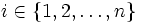$i \in \{ 1,2,\ldots, n\}$ such that$a_i \in I$. Note that the$a_i$ may be equal or distinct, i.e., some may be equal, some may be distinct.
4 product of finitely many ideals version It is a proper ideal and whenever the product of finitely many ideals is contained in it, one of the ideals is contained in it.$I$ is proper in$R$ and whenever$J_1,J_2,\ldots,J_n$ are ideals in$R$ such that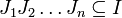$J_1J_2\ldots J_n \subseteq I$, there exists$i \in \{ 1,2,\ldots, n\}$ such that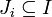$J_i \subseteq I$
5 complement saturated subset version It is a proper ideal and its complement is a multiplicatively closed saturated subset (that is, is closed with respect to the operations of multiplication and factorization).$I$ is proper in$R$ and the complement of$I$ in$R$ is a multiplicatively closed and saturated subset i.e.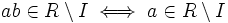$ab \in R \setminus I \iff a \in R \setminus I$ or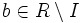$b \in R \setminus I$.
6 quotient is integral domain The quotient ring is an integral domain, i.e., a nonzero ring where the product of any two nonzero elements is nonzero. The quotient ring$R/I$ is an integral domain.

### The set of prime ideals

Further information: Spectrum of a commutative unital ring

The set of prime ideals in a commutative unital ring is termed its spectrum. The spectrum is more than just a set, it has the structure of a topological space. In fact, it is a locally ringed space.

This article defines a property of an ideal in a commutative unital ring |View other properties of ideals in commutative unital rings
This property of an ideal in a ring is equivalent to the property of the quotient ring being a/an: integral domain | View other quotient-determined properties of ideals in commutative unital rings

## Metaproperties

### Intersection-closedness

This property of ideals in commutative unital rings is not closed under taking arbitrary intersections; in other words, an arbitrary intersection of ideals with this property need not have this property

An intersection of prime ideals need not be prime. In fact, an ideal is an intersection of prime ideals iff it is a radical ideal.

### Contraction-closedness

This property of ideals in commutative unital rings is contraction-closed: a contraction of an ideal with this property, also has this property
View other contraction-closed properties of ideals in commutative unital rings

If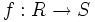$f:R \to S$ is a homomorphism of commutative unital rings, and$I$ is a prime ideal of$S$, then the contraction of$I$ to$R$, denoted$I^c$, (same as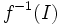$f^{-1}(I)$) is a prime ideal in$R$.

For full proof, refer: primeness is contraction-closed

Note that this implies the intermediate subring condition and the transfer condition on ideals.

### Intermediate subring condition

This property of ideals satisfies the intermediate subring condition for ideals: if an ideal has this property in the whole ring, it also has this property in any intermediate subring
View other properties of ideals satisfying the intermediate subring condition

If an ideal is prime in the whole ring, it is also prime in any intermediate subring. This is related to the fact that any subring of an integral domain is an integral domain.

### Transfer condition

This property of ideals satisfies the transfer condition for ideals: if an ideal satisfies the property in the ring, its intersection with any subring satisfies the property inside that subring

If$I$ is a prime ideal in$R$, and$S$ is any subring of$R$, then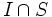$I \cap S$ is a prime ideal in$S$. Note that this implies the intermediate ring condition as well.

## Effect of property operators

### The intersection-closure

Applying the intersection-closure to this property gives: radical ideal

An ideal in a commutative unital ring is expressible as an intersection of prime ideals iff it is a radical ideal.

Further information: Intersection of prime equals radical

## In particular kinds of rings

### In rings of integers

Further information: Prime ideal in ring of integers

A ring of integers in a number field is a Dedekind domain, hence any nonzero prime ideal in this ring is a maximal ideal.

### In affine rings

Further information: Prime ideal in affine ring

Ring property assumed Nature of prime ideals
Zero-dimensional ring maximal
One-dimensional domain Zero or maximal
Principal ideal domain Zero or maximal
Dedekind domain Zero or maximal